# Solving Quadratics Quadratic Formula Discriminant Nature of the

• Slides: 50Solving Quadratics Quadratic Formula Discriminant – Nature of the Roots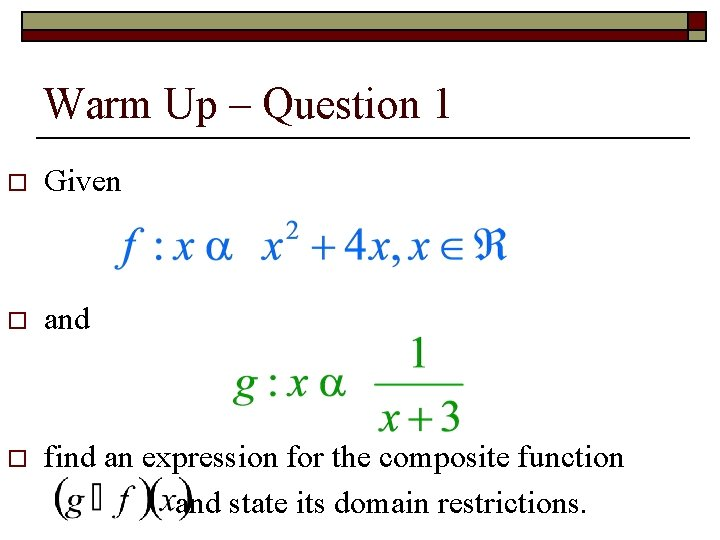Warm Up – Question 1 o Given o and o find an expression for the composite function and state its domain restrictions.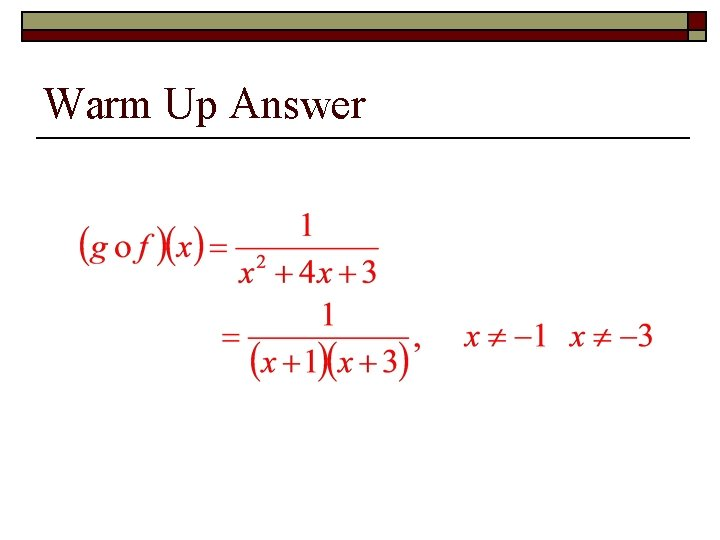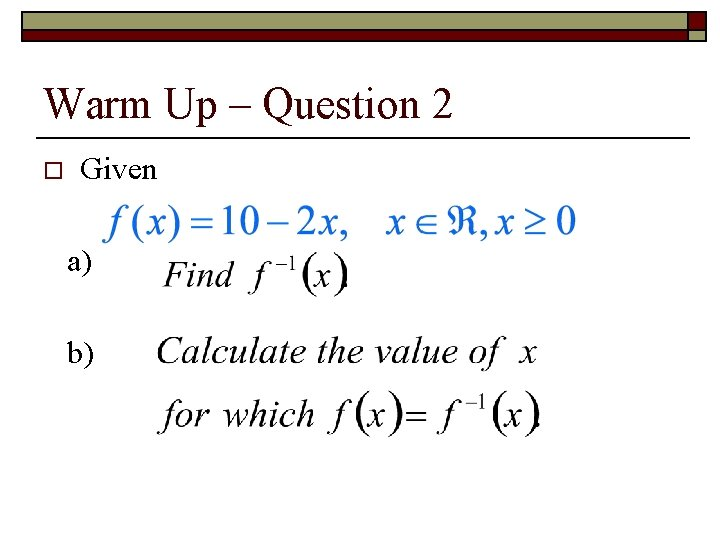Warm Up – Question 2 o Given a) b)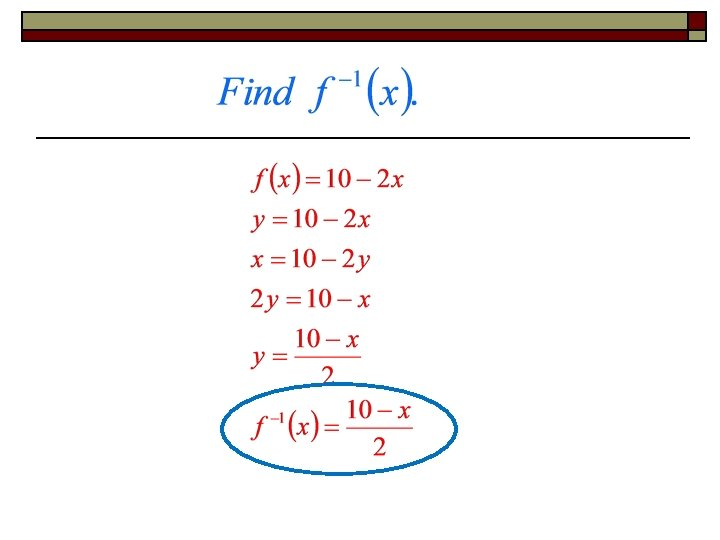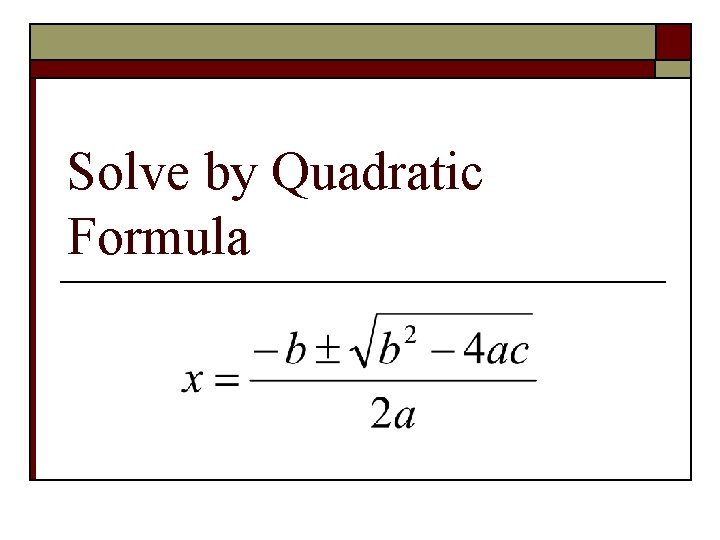Solve by Quadratic FormulaDerive the Quadratic Formula by Completing the Square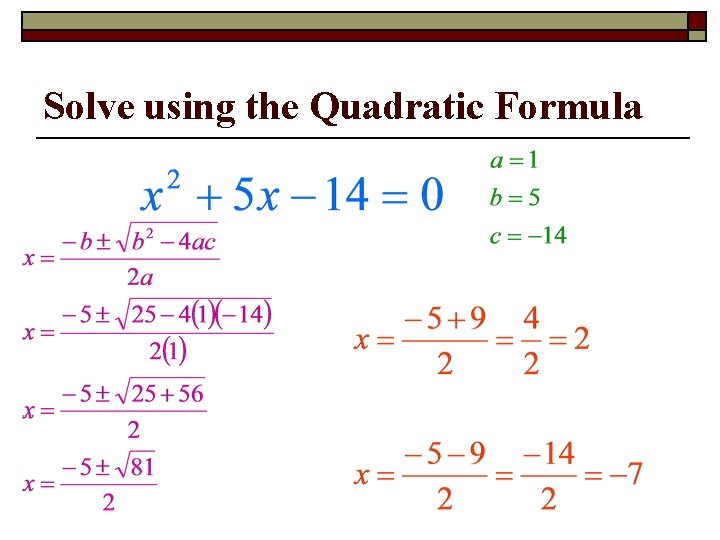Solve using the Quadratic Formula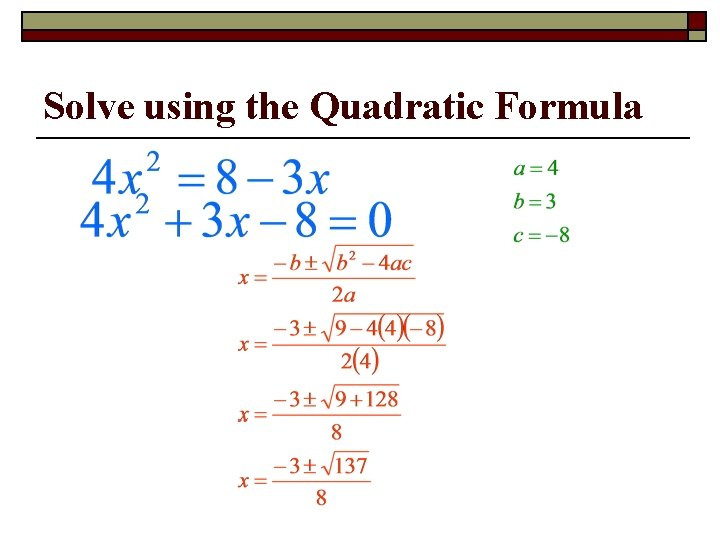Solve using the Quadratic Formula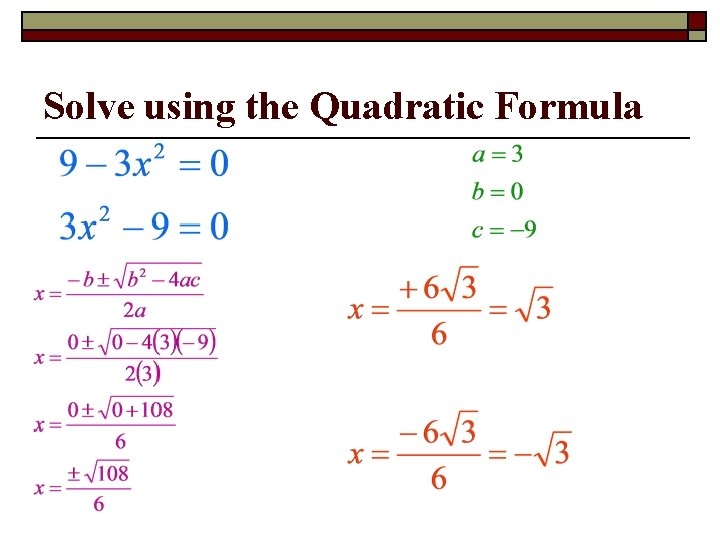Solve using the Quadratic Formula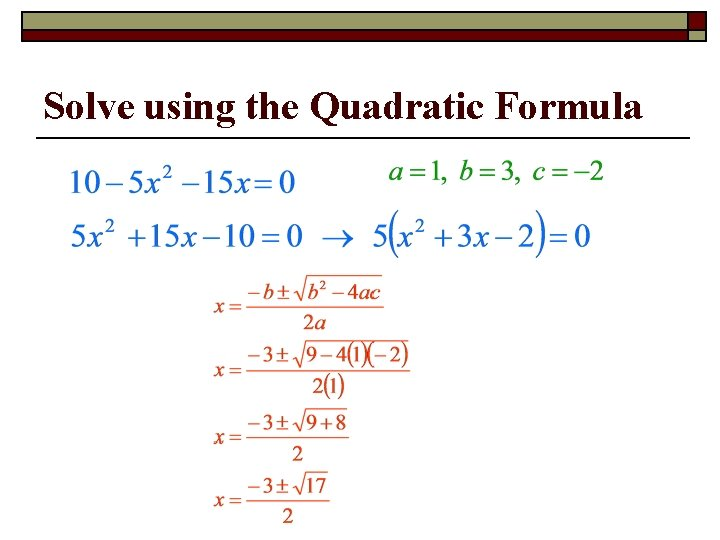Solve using the Quadratic Formula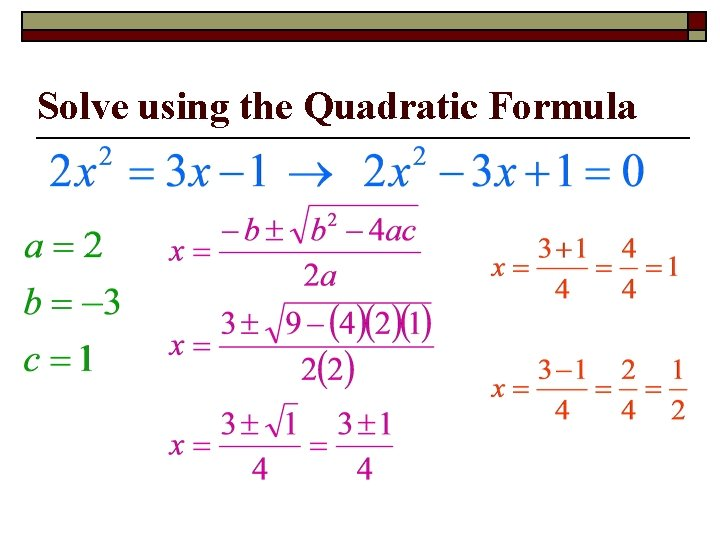Solve using the Quadratic FormulaSolve using the Quadratic Formula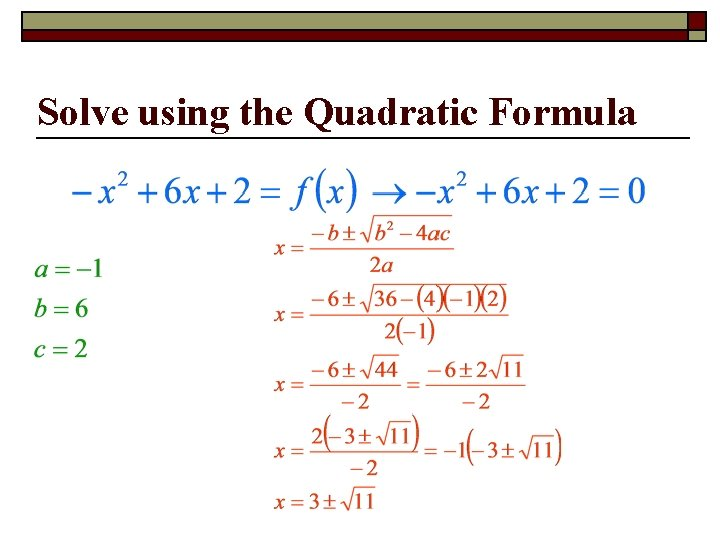Solve using the Quadratic Formula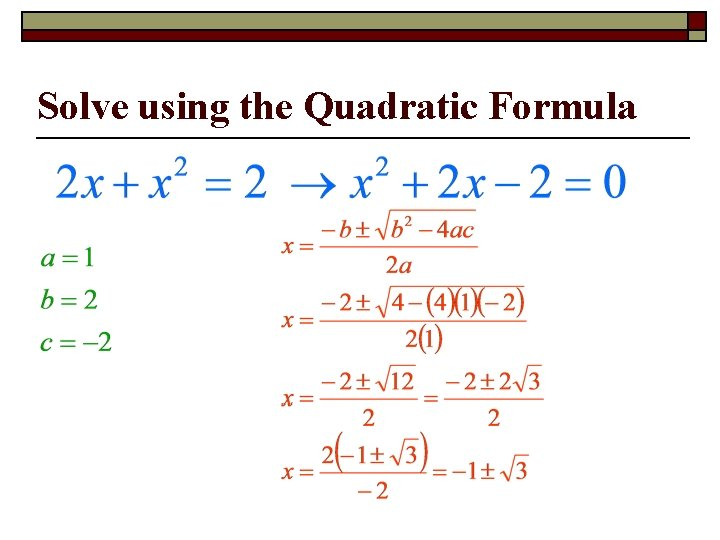Solve using the Quadratic Formula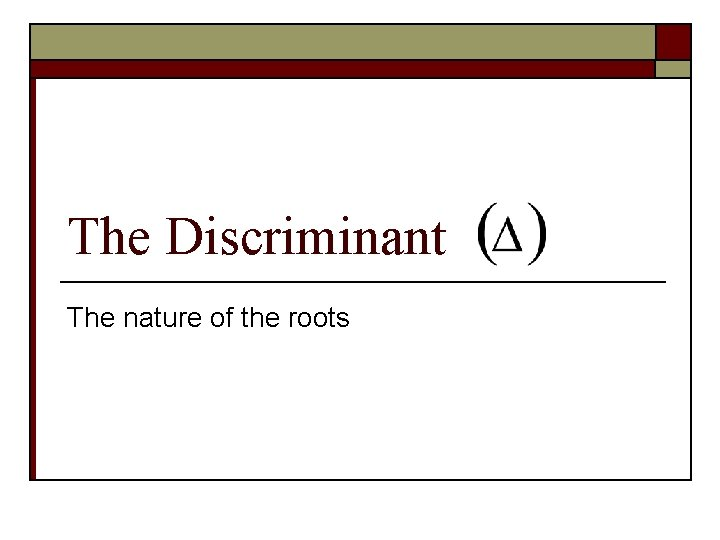The Discriminant The nature of the rootsThe Discriminant o o It comes from the quadratic formula. =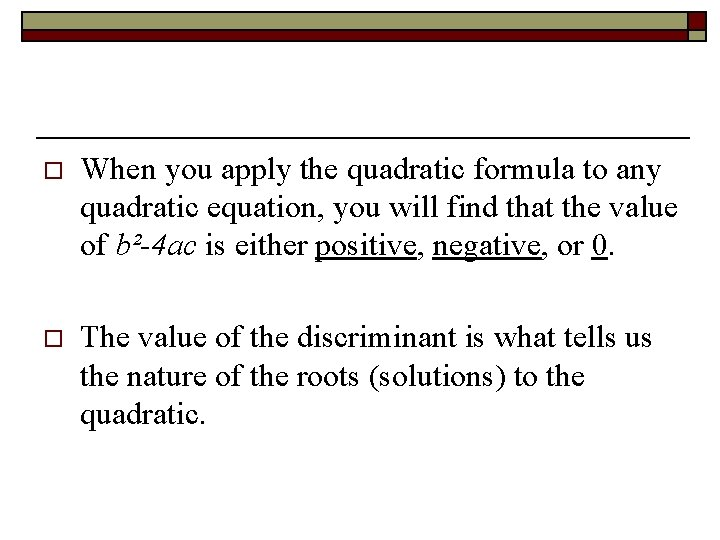o When you apply the quadratic formula to any quadratic equation, you will find that the value of b²-4 ac is either positive, negative, or 0. o The value of the discriminant is what tells us the nature of the roots (solutions) to the quadratic.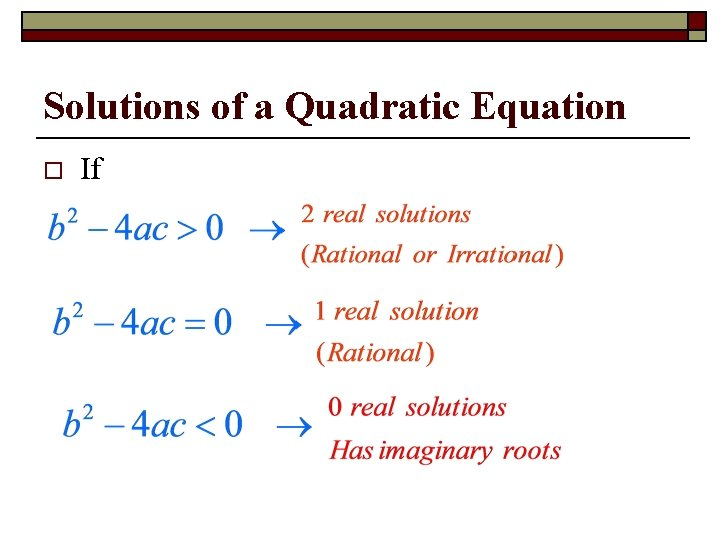Solutions of a Quadratic Equation o If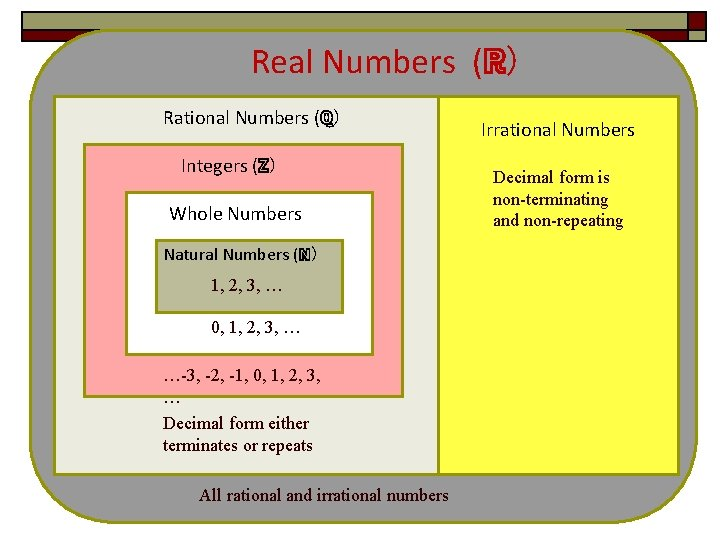Real Numbers (ℝ) Rational Numbers (ℚ) Integers (ℤ) Whole Numbers Natural Numbers (ℕ) 1, 2, 3, … 0, 1, 2, 3, … …-3, -2, -1, 0, 1, 2, 3, … Decimal form either terminates or repeats All rational and irrational numbers Irrational Numbers Decimal form is non-terminating and non-repeating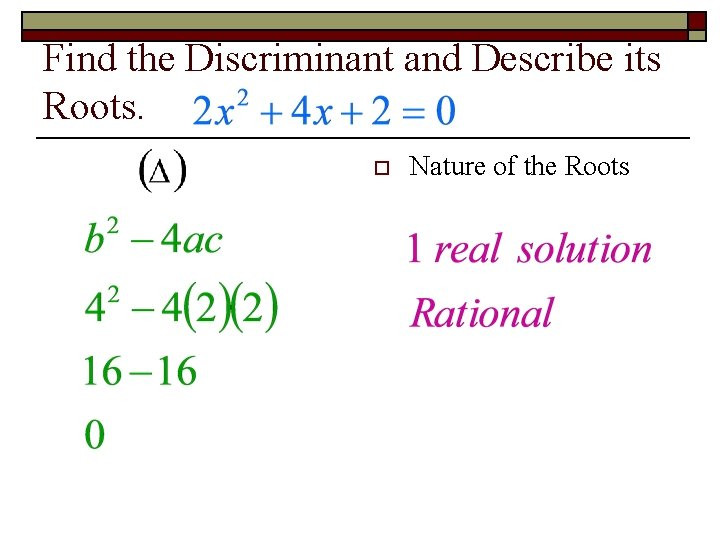Find the Discriminant and Describe its Roots. o Nature of the RootsFind the Discriminant and Describe its Roots. o Nature of the RootsFind the Discriminant and Describe its Roots o Nature of the Roots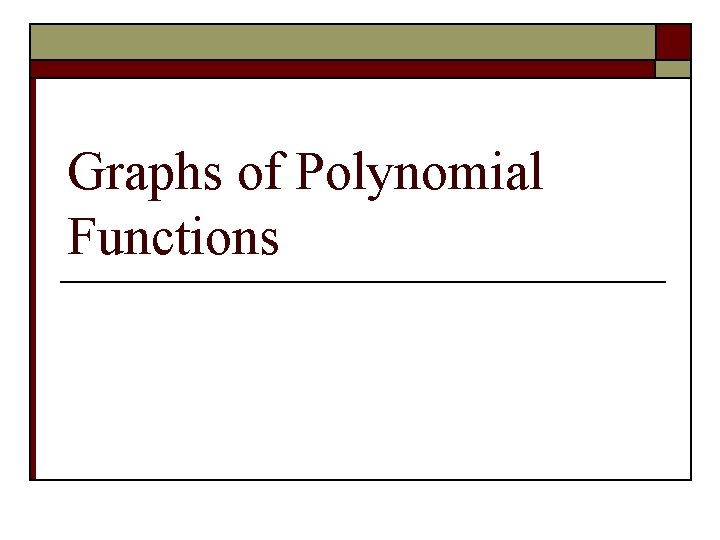Graphs of Polynomial FunctionsExplore – Look at the relationship between the degree & sign of the leading coefficient and the right- and left-hand behavior of the graph of the function.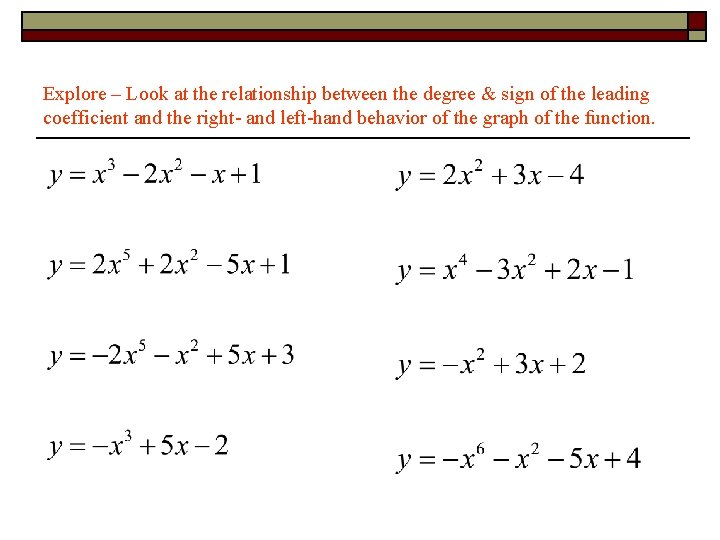Explore – Look at the relationship between the degree & sign of the leading coefficient and the right- and left-hand behavior of the graph of the function.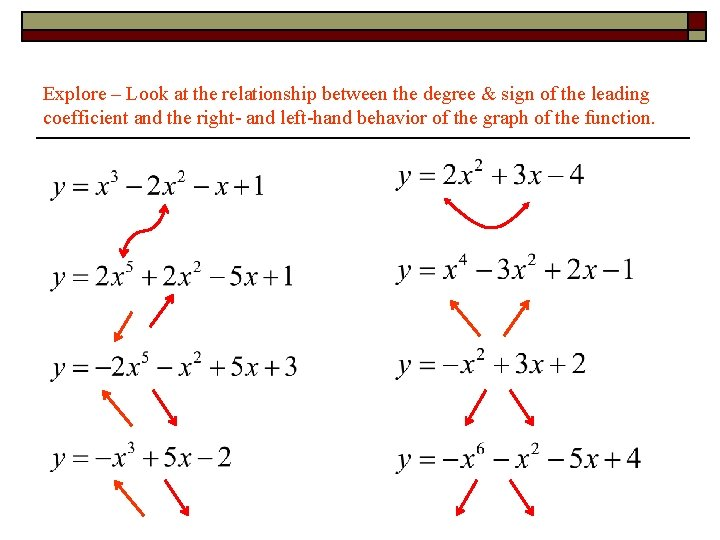Explore – Look at the relationship between the degree & sign of the leading coefficient and the right- and left-hand behavior of the graph of the function.Continuous Function o A function is continuous if its graph can be drawn with a pencil without lifting the pencil from the paper. Continuous Not ContinuousPolynomial Function o Polynomial Functions have continuous graphs with smooth rounded turns. o Written: o Example:Explore using graphing Calculator Describe graph as S or W shaped. Function Degree # of U turnsGeneralizations? o The number of turns is one less than the degree. o Even degree → “W” Shape o Odd degree → “S” ShapeDescribe the Shape and Number of Turns.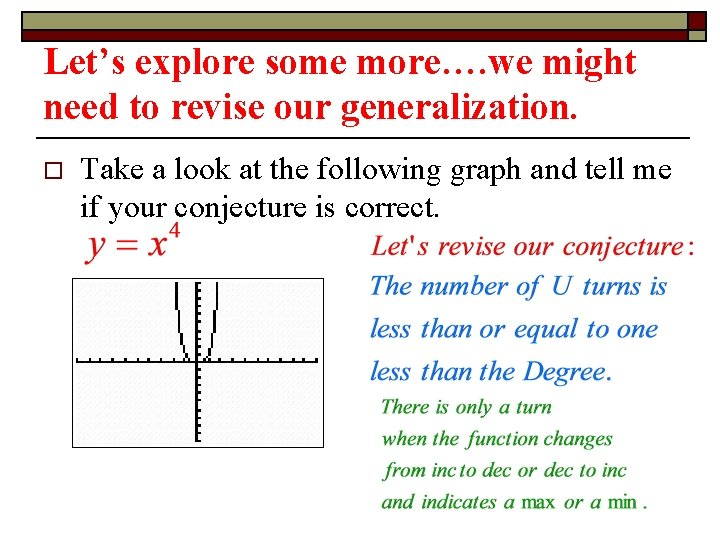Let’s explore some more…. we might need to revise our generalization. o Take a look at the following graph and tell me if your conjecture is correct.Lead Coefficient Test When n is odd Lead Coefficient is Positive: (an >0), the graph falls to the left and rises to the right Lead Coefficient is Negative: (an <0), the graph rises to the left and falls to the rightLead Coefficient Test When n is even Lead Coefficient is Positive: (an >0), the graph rises to the left and rises to the right Lead Coefficient is Negative: (an <0), the graph falls to the left and falls to the rightLeading Coefficient: an End Behavior - a>0 a<0 left right n - even n - oddUse the Leading Coeffiicent Test to describe the right-hand left-hand behavior of the graph of each polynomial function: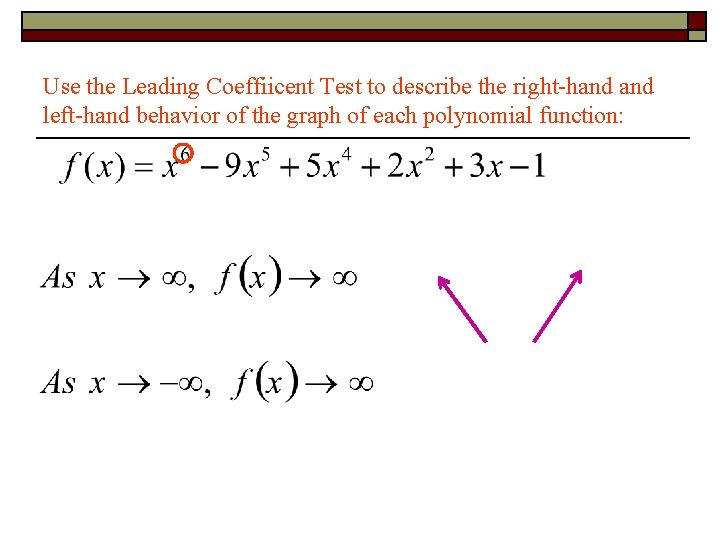Use the Leading Coeffiicent Test to describe the right-hand left-hand behavior of the graph of each polynomial function: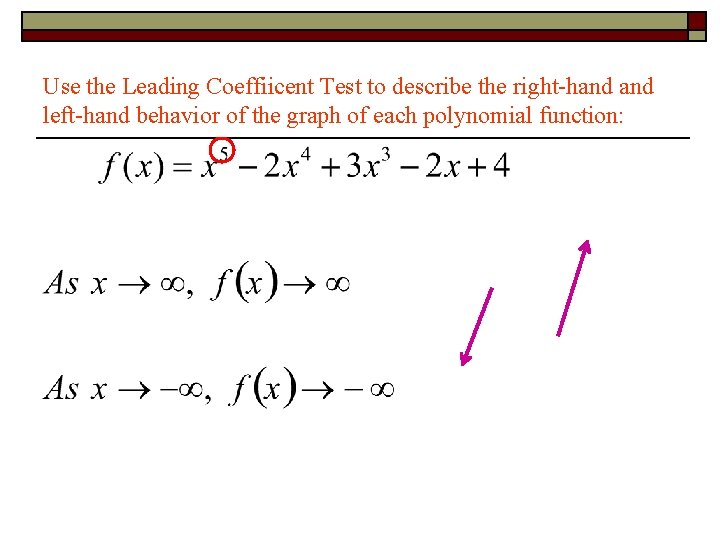Use the Leading Coeffiicent Test to describe the right-hand left-hand behavior of the graph of each polynomial function:Use the Leading Coeffiicent Test to describe the right-hand left-hand behavior of the graph of each polynomial function:A polynomial function (f) of degree n , the following are true o The function has at most n real zeros o The graph has at most (n-1) relative extrema (relative max/min)Local Max / Min (in terms of y) Increasing / Decreasing (in terms of x)Local Max / Min (in terms of y) Increasing / Decreasing (in terms of x)Approximate any local maxima or minima to the nearest tenth. Find the intervals over which the function is increasing and decreasing.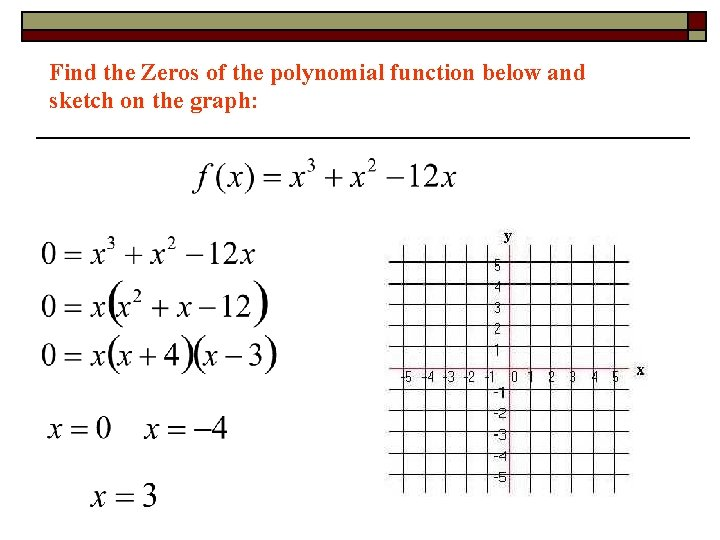Find the Zeros of the polynomial function below and sketch on the graph: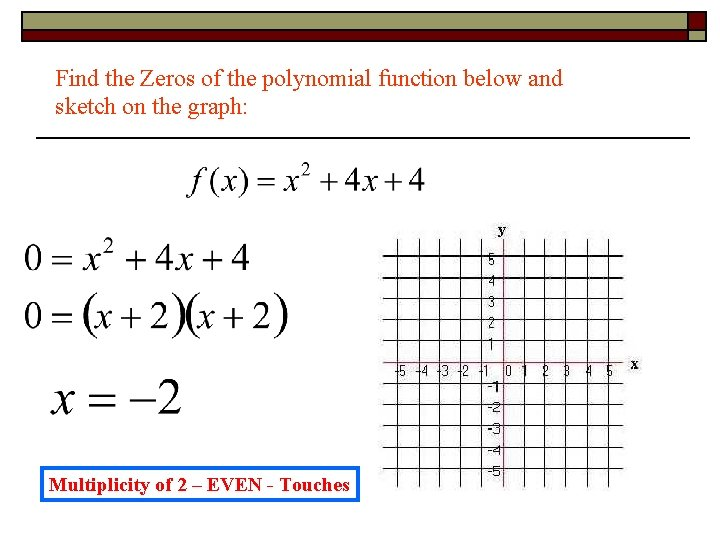Find the Zeros of the polynomial function below and sketch on the graph: Multiplicity of 2 – EVEN - Touches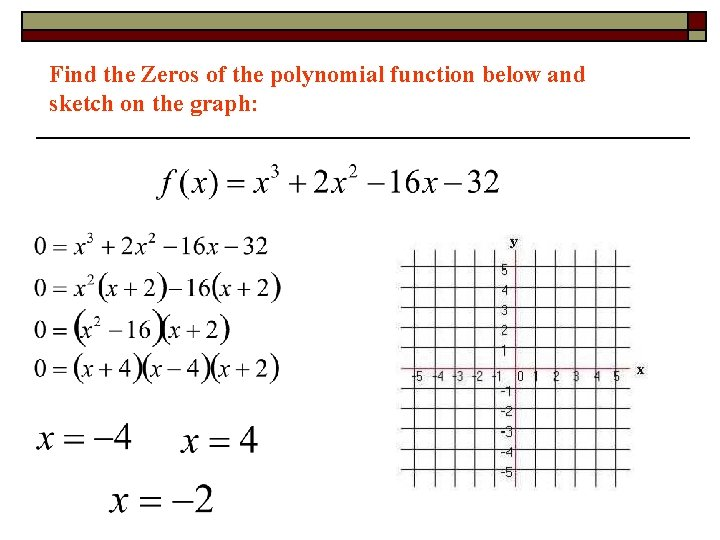Find the Zeros of the polynomial function below and sketch on the graph: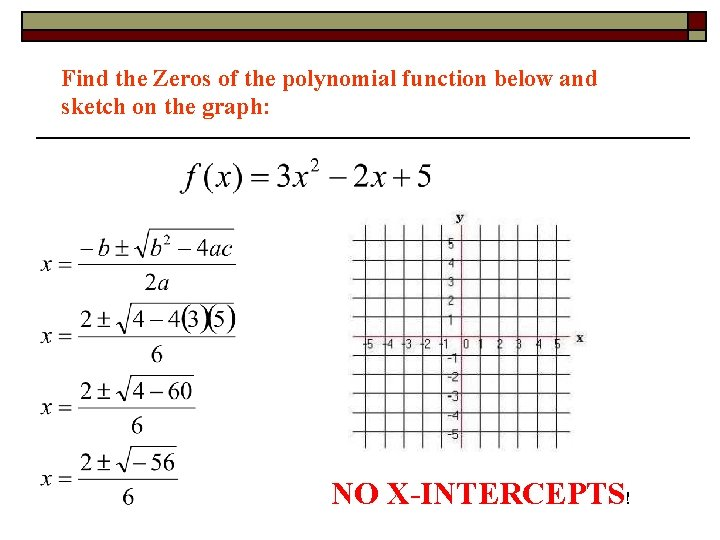Find the Zeros of the polynomial function below and sketch on the graph: NO X-INTERCEPTS!Find the Zeros of the polynomial function below and sketch on the graph: